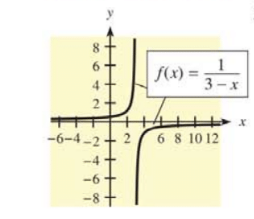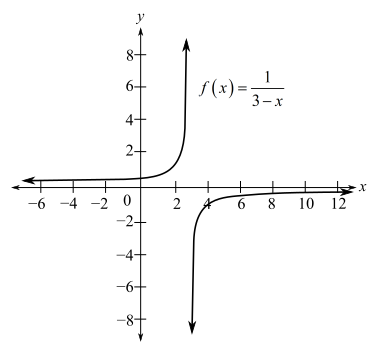Chapter 12.3, Problem 35E### Mathematical Applications for the ...

11th Edition
Ronald J. Harshbarger + 1 other
ISBN: 9781305108042

#### Solutions

Chapter
Section### Mathematical Applications for the ...

11th Edition
Ronald J. Harshbarger + 1 other
ISBN: 9781305108042
Textbook Problem

# In Problems 35 and 36, a function f ( x ) and its graph are given. Find the family f ( x )   =   ∫ f ( x )   d x and graph the member that satisfies F ( 0 )   =   0 .To determine

To calculate: The family of F(x)=f(x)dx and to graph the member that satisfies F(0)=0 if the function f(x) and its graph is provided as:Explanation

Given information:

The provided graph is:

And, the provided function is, f(x)=13x.

Calculation:

Integrate the provided function as:

13xdx=ln|3x|+C

It is provided that F(x)=f(x)dx and it is also provided that F(0)=0.

0=ln|30|+CC=ln|3|

Now substitute the value of C as:

F(x)=ln|3|ln|3x|

Hence, the family of F(x)=f(x)dx is, F(x)=ln|3|ln|3x|

### Still sussing out bartleby?

Check out a sample textbook solution.

See a sample solution

#### The Solution to Your Study Problems

Bartleby provides explanations to thousands of textbook problems written by our experts, many with advanced degrees!

Get Started

#### Find more solutions based on key concepts